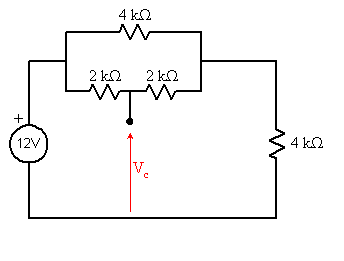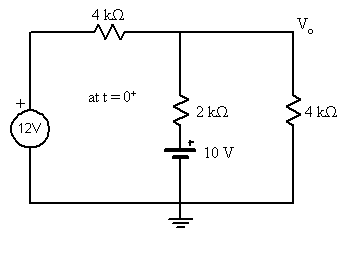# Capacitor Resistors problem, , exam preparation

Capacitor Resistors problem, URGENT PLZ, exam preparation

## Homework Statement

In the following transient circuit, assume at t<0, the circuit is at steady state.
Assume vc(t) is the voltage across the capacitor and v0 (t) is the output voltage as shown:
Find (a) vc(0+) (b) vc(∞) (c) vc(t) and (d) v0(t) for t>0
http://sphotos-b.xx.fbcdn.net/hphotos-ash4/c0.0.299.299/p403x403/381831_509320622420791_1588549456_n.jpg [Broken]

## The Attempt at a Solution

I am pretty first stuck right at the beginning
A)
For this part, the capacitor will act as "open", so I remove the capacitor.

But then I have no idea how to find the voltage.

B) After the switch is opened for ∞, we can again open the capacitor.
the 2K on the left will be out of the circuit as well?

so the voltage of the capacitor will be the same as the 4K on the right side?

the current will not go through the 2k in the middle?

if so, the voltage will be 6V?

C)we need V(0) for this and time constant. τ = c/Rth
to find Rth we remove all source and take out the capacitor.
and again, I am not sure if the 2K resistors will be in series?
if they are then just take the equilvalent resistor
[(2+2)|| 4]+4 = 6k ?
and then just plug into the Vc(t) = (V(0) - V(∞)) e(-t/T) + V(∞)

D) Is Vo(t) = Vc(t) for t>0
since Vo(t) = V of 4K?

Thanks for your time

Last edited by a moderator:

gneill
Mentor

## Homework Statement

In the following transient circuit, assume at t<0, the circuit is at steady state.
Assume vc(t) is the voltage across the capacitor and v0 (t) is the output voltage as shown:
Find (a) vc(0+) (b) vc(∞) (c) vc(t) and (d) v0(t) for t>0
http://sphotos-b.xx.fbcdn.net/hphotos-ash4/c0.0.299.299/p403x403/381831_509320622420791_1588549456_n.jpg [Broken]

## The Attempt at a Solution

I am pretty first stuck right at the beginning
A)
For this part, the capacitor will act as "open", so I remove the capacitor.

But then I have no idea how to find the voltage.
Just rearrange the layout a bit and I think you'll find an approach:B) After the switch is opened for ∞, we can again open the capacitor.
the 2K on the left will be out of the circuit as well?

so the voltage of the capacitor will be the same as the 4K on the right side?

the current will not go through the 2k in the middle?

if so, the voltage will be 6V?
That looks fine. Proceed with the knowledge that the middle 2k resistor is "disappeared" by the opening of the switch.

#### Attachments

Last edited by a moderator:

thanks for your response. can you check my answers please. Follow that approach I get
a) Vc(0) = 10V

b)Vc(∞) = 6V

c) Vc(t) = (10-6)e^(-2.5t) + 6

d) Vc(t) =Vo(t) ??

Thanks for your time

gneill
Mentor

thanks for your response. can you check my answers please. Follow that approach I get
a) Vc(0) = 10V

b)Vc(∞) = 6V

c) Vc(t) = (10-6)e^(-2.5t) + 6

d) Vc(t) =Vo(t) ??

Thanks for your time

Looks okay except for (d). Draw the equivalent circuit for time > 0. Initially the capacitor begins with its initial voltage of 10V from part (a). So pretend it's a voltage source at the instant t = 0+. What then is Vo initially?

It seem to me that if we go on to find Vo(0+) we then have to deal with superposition since there are 2 voltage source?

actually in the problem statement, there is a hint for part d, which says apply KCL at top node.

and I dont really know what to do with that hint and how to use equation from Vc(t) to find Vo(t)

=(

gneill
Mentor

It seem to me that if we go on to find Vo(0+) we then have to deal with superposition since there are 2 voltage source?

actually in the problem statement, there is a hint for part d, which says apply KCL at top node.

and I dont really know what to do with that hint and how to use equation from Vc(t) to find Vo(t)

=(

Draw the circuit for the instant that the switch opens, replacing the capacitor with a voltage source of value equal to the initial capacitor potential:Use whatever circuit analysis techniques you want to find the initial value for Vo. One method is to use nodal analysis (KCL) at the output node. Similarly, find Vo for time >> 0. These two endpoints are connected by an exponential function with the same time constant as the capacitor voltage function.